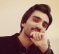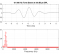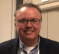Started by 4 years ago6 replieslatest reply 4 years ago162 views

Hi everyone!

I am solving a computer exercise in Andrea Goldsmith's Wireless Communication book. The problem statement is: "the simulation first generates a white noise process and then passes it through a first-order filter with a pole at exp(−d / Xc). Assume Xc = 20 m and plot the resulting log-normal fading process over a distance d ranging from 0 m to 200 m, sampling the process every meter."

I know that the white noise process is just randn(1,200) in MATLAB.

Now my problem is since d is ranging from 0 to 200 (which is d = 0:200 in MATLAB) how do I filter? I mean the pole is changing since d is not fixed...I am completely clueless here.

Regards

Shahbaz

[ - ]I think all the information is stated in the question.  Consider,

close all; clear; clc;
set(0, 'DefaultFigureWindowStyle', 'docked');

sampleRate_meters=1;
d_meters=0:1:199;

a=exp(-sampleRate_meters/20);
b=1-a; a=[1 -a];

figure; freqz(b, a, 4096, sampleRate_meters)
figure; zplane(b, a);

x=randn(1, 200);
y=filter(b, a, x);

figure; plot(d_meters, x); hold on; plot(d_meters, y, 'r'); grid on; shg; ...
xlabel('Distance (meters)'); ylabel('Amplitude (unitless)'); title('Filtered White-noise Process');


See Exponential Filter section.

[ - ]Hi MichaelRW, thanks for your answer and for writing the code for me. But the book's text (section 2.7) and exercise problem 2.20 both state that we need to pass the white noise process through a filter with a pole at exp(-delta/Xc) where Xc=20 and delta is what I denoted by d in the problem statement (i.e., delta = 0:200).

I don't understand why you used exp(-samplerate_meters/Xc).

[ - ]Hello,

I don't think I fully understand the question.  Having said that the only thing I can suggest, having read Rick's post, is applying the set of 201 filters to a ensemble of random-noise signals and summing the filtered noise signals.

Michael.

[ - ]Hi Shabaz.
I share your uncertainty here. If you must pass a
random-noise signal through a filter having a pole
at z = exp(-d/20), and 'd' ranges from 0 -to- 200,
then it seems like you'll be implementing 201 different
filters! And that doesn't make sense to me.

Sorry I can't be of help here. I'm posting my
Reply here only to let you know I sympathize with

[-Rick-]

[ - ]Hi Rick!

Well...it's a bit comforting to know that the author whose book I read in undergrad is also puzzled. :)

[ - ]